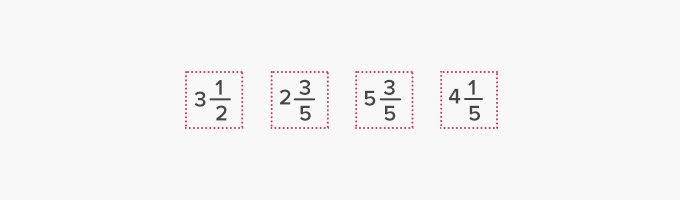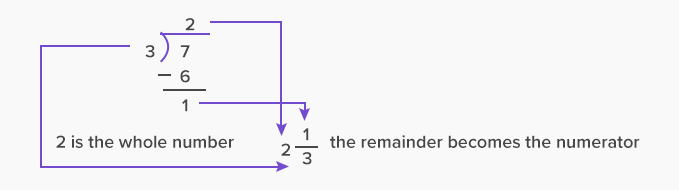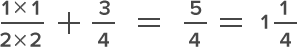# Mixed Number - Definition with Examples

The Complete K-5 Math Learning Program Built for Your Child

• 30 Million Kids

Loved by kids and parent worldwide

• 50,000 Schools

Trusted by teachers across schools

• Comprehensive Curriculum

Aligned to Common Core

## What are Mixed Numbers?

A mixed number is a whole number, and a proper fraction represented together.  It generally represents a number between any two whole numbers.

Look at the given image, it represents a fraction that is greater than 1 but less than 2. It is thus, a mixed number.Some other examples of mixed numbers areParts of a mixed number

A mixed number is formed by combining three parts: a whole number, a numerator, and a denominator. The numerator and denominator are part of the proper fraction that makes the mixed number.Properties of mixed numbers

• It is partly a whole number.

• It is partly a fraction.

Converting improper fractions to mixed fractions.

Step 1: Divide the numerator by the denominator.

Step 2: Write down the quotient as the whole number.

Step 3: Write down the remainder as the numerator and the divisor as the denominator.

For example, we follow the given steps to convert 7/3 into a mixed number form.

Step 1: Divide 7 by 3Step 2: Write quotient, divisor and remainder in form as in step 2 and step 3 above.One can add mixed numbers by rearranging the whole numbers, adding them separately and adding the leftover fractions individually and in the end combing them all.

1+ 3 34

Adding the whole numbers separately and the fractions separately.

For whole numbers:

1+3 = 4

For fractions:  Find the LCM and then addIn the end, adding both the parts together.

4+1 14 =5 14

Real life examples

We can check our understanding of mixed fractions by expressing the parts of a whole as mixed fractions while serving a pizza or a pie at home. Leftover pizzas, half-filled glasses of milk form examples of mixed fractions.Fun Facts Mixed numbers are also called mixed fractions.

Won Numerous Awards & Honors